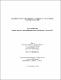## An improved implementation of sparsity detection of sparse derivative matrices2018
##### Authors
Jesmin, Tasnuba
University of Lethbridge. Faculty of Arts and Science
##### Publisher
Lethbridge, Alta. : Universtiy of Lethbridge, Department of Mathematics and Computer Science
##### Abstract
Optimization is a crucial branch of research with application in numerous domain. Determination of sparsity is a vital stream of optimization research with potentials for improvement. Manual determination of sparsity structure of Jacobian matrix for a large problem is complicated and highly error-prone. The main motivation of this research is to propose an efficient algorithm which can effectively detect and represent sparsity of unknown Jacobian matrices. Automated sparsity detection algorithms find an optimal or near-optimal solution, which reduces time and space complexity for large scale data. Our proposed approach efficiently generates symmetric pattern utilizing band matrix and reduces the number of gradient evaluation. For efficient solution, we integrate our approach with existing pattern detection process. Greedy coloring algorithm is used for column portioning and multilevel algorithm with voting scheme is implemented for detection of sparsity pattern. Finally, parallel computation is used to reduce processing time of the overall approach.
##### Keywords
Jacobians , Combinatorial optimization , Sparse matrices -- Data processing , Graph coloring , Parallel programs (Computer programs) , Matix devrivatives , sparse data structure , CPR algorithm , sparse derivative matrices , Jacobian matrix , multilevel algorithm , parallel implementation